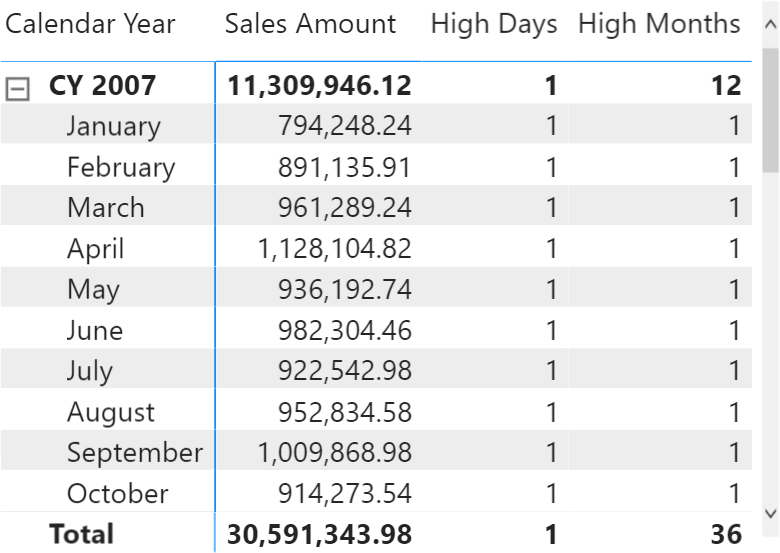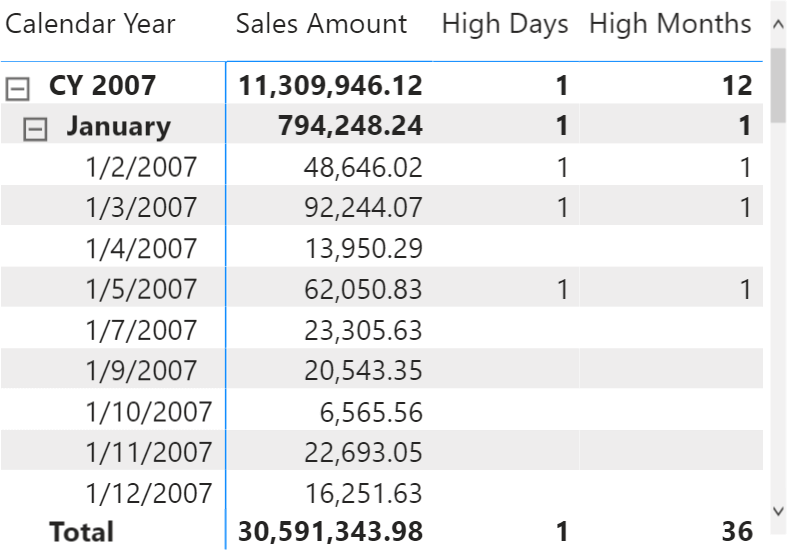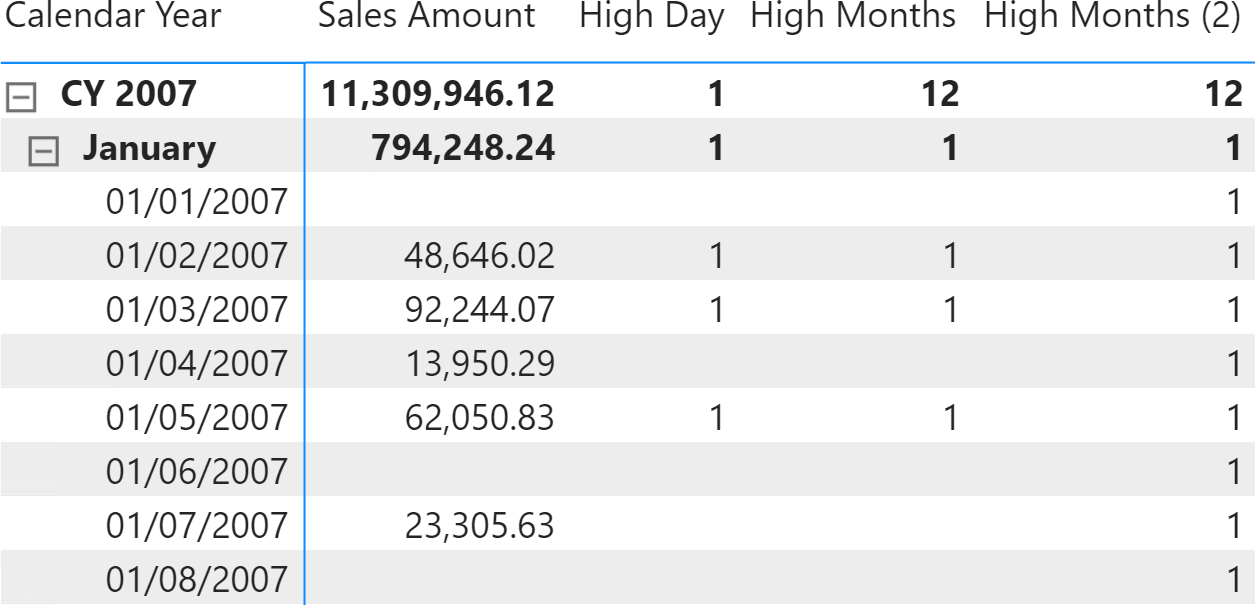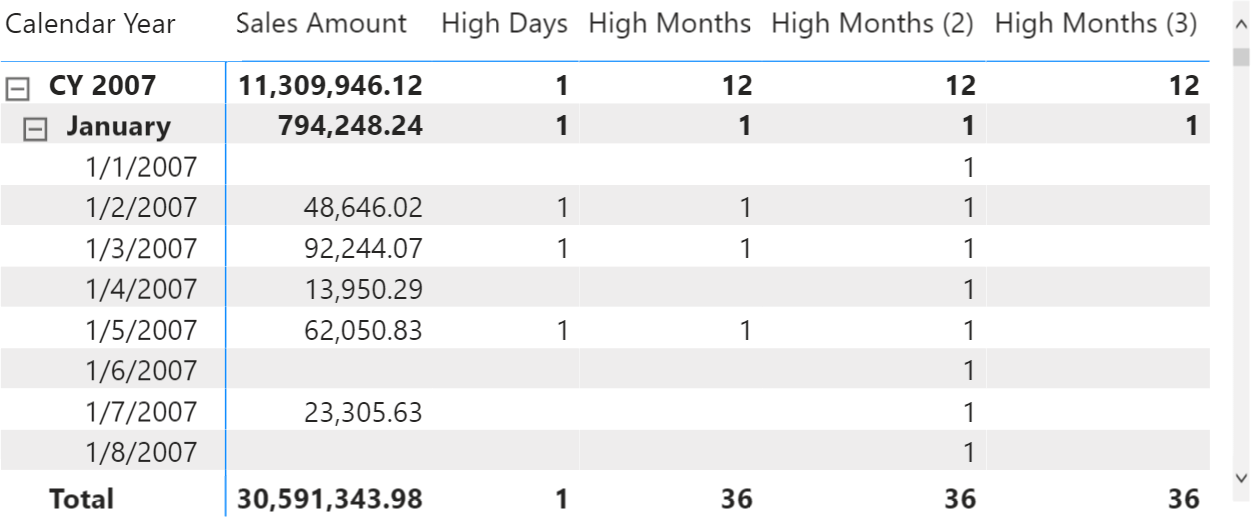In the article Obtaining accurate totals in DAX, we described the granularity of a formula as being the level of detail that the formula uses to compute its result. If you are not familiar with formula granularity, please read that article first, because this is the natural continuation.

The starting point is a measure computing the number of months where Sales Amount is greater than 30,000:

```High Months =
SUMX (
VALUES ( 'Date'[Calendar Year Month] ),
IF ( [Sales Amount] > 30000, 1 )
)
```

In a report at the month level, the formula produces the expected results.Nevertheless, when expanded at the date level that same formula produces incorrect figures. As you can see in the next figure, the High Months measure returns 1 for those days where Sales Amount is greater than 30,000.The formula is designed to work at the level of the month and above – like quarter and year. Below the month level – like day – the results are inaccurate. At this point, the important question is what the result below the granularity of the formula should be. There are several possible answers; all of them are sort-of correct:

1. It is fine as it is, no need to fix it; users should not use the measure at the day level.
2. It always needs to show 1, because each individual date belongs to a month that satisfies the condition.
3. It must show a blank, because that granularity is not supported.

If you are ok with the first option, then the remaining part of the article is useless. Still, you are quitting the game too early: sooner than later, a user will use and read the values at the unsupported granularity. A real DAX pro does not let their formulas compute an incorrect value. Under. Any. Circumstances.

The second option, which proves to be much better than the first one, requires some deep reasoning about the filter context. For example, when the report is showing data at the day level, the measure must move the granularity of the calculation to the corresponding month level by removing the filter on the day column, while keeping the filter on the month only. The right way to obtain this behavior is by using ALLEXCEPT in CALCULATE:

```High Months (2) =
SUMX (
VALUES ( 'Date'[Calendar Year Month] ),
CALCULATE (
IF ( [Sales Amount] > 30000, 1 ),
ALLEXCEPT ( 'Date', 'Date'[Calendar Year Month] )
)
)
```ALLEXCEPT removes any filter from the Date table, except the filter that is currently being created by the context transition invoked by CALCULATE during the iteration over the months. Please note that in this case ALLEXCEPT is the correct solution – though we often suggest you use ALL/VALUES instead. Indeed, this version of the formula would not work:

```High Months (Wrong) =
SUMX (
VALUES ( 'Date'[Calendar Year Month] ),
CALCULATE (
IF ( [Sales Amount] > 30000, 1 ),
ALL ( 'Date' ),
VALUES ( 'Date'[Calendar Year Month] )
)
)
```

The reason why this formula is wrong is quite subtle: when VALUES is being evaluated, the context transition still has not taken place. Therefore, the filter on the year/month is not effective yet. This is not an issue at the month or day level inside the matrix, but it provides an incorrect result at the grand total level, where no filter is active. ALLEXCEPT being a modifier, it executes after the context transition; it efficiently removes any filter except the one on Date[Calendar Year Month] introduced by the context transition.

The third and last option requires blanking the value when the granularity of the report is below the granularity of the formula. The DAX code is not too complicated, but the reasoning behind it requires some special attention.

The formula must detect the granularity of the cell in the report before completing the calculation. If the cell is below the supported granularity, the result must be a blank. In order to detect the granularity of the cell, we can compare the number of rows in the Date table at the cell granularity (the filter context of the cell being evaluated) with the supported granularity (the granularity defined by the formula).

Because the formula should work at the month granularity, we create two variables. The DaysAtCurrentGrain variable contains the number of visible rows. The DaysAtFormulaGrain variable contains the number of rows in a new filter context where we enforce the month granularity. If the two variables return the very same number, then DAX is working in a filter context at the month granularity level or above that (such as the year). If the two numbers are different, it means that the formula is computing the result below the supported granularity, and the result must be a blank:

```High Months (3) =
VAR DaysAtCurrentGrain =
COUNTROWS ( 'Date' )
VAR DaysAtFormulaGrain =
CALCULATE (
COUNTROWS ( 'Date' ),
ALL ( 'Date' ),
VALUES ( 'Date'[Calendar Year Month] )
)
VAR IsSafeToCompute = (DaysAtCurrentGrain = DaysAtFormulaGrain)
VAR Result =
IF (
IsSafeToCompute,
SUMX (
VALUES ( 'Date'[Calendar Year Month] ),
IF ( [Sales Amount] > 30000, 1 )
)
)
RETURN
Result
```

The High Months (3) measure returns a blank whenever the report goes below the supported granularity – guaranteeing that if a number is shown, it is the correct one.As you can see, computing the right values at any granularity proves to be challenging. Building useful measures in a data model always requires thinking in terms of granularity and accuracy of the results.

DAX offers all the tools that are necessary to obtain the correct result. It is up to you to think in advance about the conditions under which your formula might fail. Once you have identified these critical conditions, you must define what the expected result at the given granularity is, and eventually implement the solution in DAX.

ALLEXCEPTCALCULATE modifier

Returns all the rows in a table except for those rows that are affected by the specified column filters.

`ALLEXCEPT ( <TableName>, <ColumnName> [, <ColumnName> [, … ] ] )`

CALCULATEContext transition

Evaluates an expression in a context modified by filters.

`CALCULATE ( <Expression> [, <Filter> [, <Filter> [, … ] ] ] )`

VALUESWhen a column name is given, returns a single column table of unique values. When a table name is given, returns a table with the same columns and all the rows of the table (including duplicates) with the additional blank row if present.

`VALUES ( <TableNameOrColumnName> )`